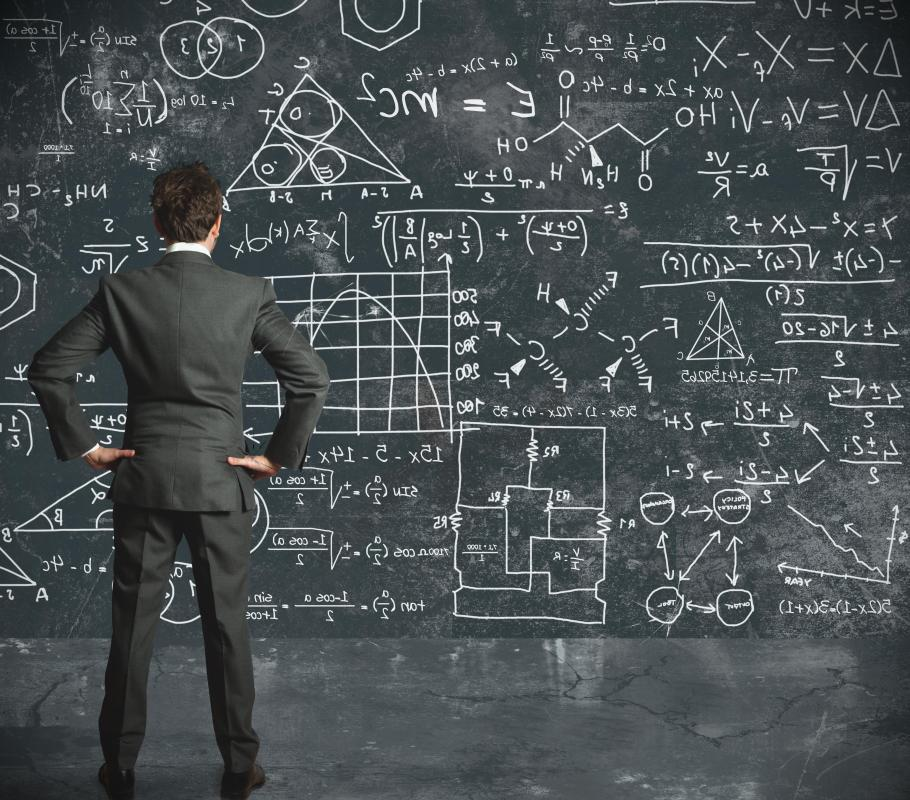# What Is Reduced Mass?

Paul Reed

When looking at particles as small as an atom, or objects as large as the Earth and moon, reduced mass can be an important consideration when calculating the behavior of the objects moving around each other. A proton and an electron or the Earth and Moon circle each other with widely different mass, or the amount of each object affected by the force of gravity. Using a reduced mass equation can simplify the calculations of how each will behave in different situations.Reduced mass can be an important consideration when calculating the behavior of the objects moving around each other.

As two objects revolve around each other, they have a force that can be calculated by Sir Isaac Newton's second law of mechanics, that calculates the forces between objects based on their mass and distance. Newton (1642-1727) was a mathematician, chemist and physicist who formulated many concepts on planetary motion and gravity. His second law describes the forces that occur between two objects, but assumes that the objects are stationary. Reduced mass takes into account each object and their distance from each other, giving a value that can be used in Newton's equation and other calculations for gravity and acceleration.

The Earth and Moon have widely different sizes, and it might be assumed that the Earth is the center of rotation of the two bodies. This is not exactly true, because the Moon affects the rotation point, called the center of rotation, due to its distance from the Earth and its mass. Using the center of the Earth would create errors in the calculation if not corrected for the Moon's mass.

Reduced mass is calculated from the mass of both objects multiplied together, then divided by the sum of the mass of the two objects. The result can then be used to calculate force and gravity effects as though there was a single mass at a point called the center of rotation. An example of this is connecting two balls with a rope, with the balls having different weights. Attempting to spin the balls by holding the rope in the middle would be unsuccessful. The experimenter would have to hold the rope closer to the heavier ball, which is the center of rotation of the two balls.

Calculations for reduced mass can also be used for small atomic particles. When electrons spin around an atomic nucleus, they create a center of mass and rotation at points other than the center of the nucleus. Solving for the reduced mass creates values that can then be used for other molecular forces.

## You might also Like